# PSAT Math : How to find the volume of a cone

## Example Questions

### Example Question #863 : Geometry

An empty tank in the shape of a right solid circular cone has a radius of r feet and a height of h feet. The tank is filled with water at a rate of w cubic feet per second. Which of the following expressions, in terms of r, h, and w, represents the number of minutes until the tank is completely filled?

20w/(π(r2)(h))

π(r2)(h)/(180w)

180w/(π(r2)(h))

π(r2)(h)/(20w)

π(r2)(h)/(60w)

π(r2)(h)/(180w)

Explanation:

The volume of a cone is given by the formula V = (πr2)/3. In order to determine how many seconds it will take for the tank to fill, we must divide the volume by the rate of flow of the water.

time in seconds = (πr2)/(3w)

In order to convert from seconds to minutes, we must divide the number of seconds by sixty. Dividing by sixty is the same is multiplying by 1/60.

(πr2)/(3w) * (1/60) = π(r2)(h)/(180w)

### Example Question #1 : Cones

A cone has a base radius of 13 in and a height of 6 in.  What is its volume?

1352π in3

4394π in3

1014π in3

338π in3

338π in3

Explanation:

The basic form for the volume of a cone is:

V = (1/3)πr2h

For this simple problem, we merely need to plug in our values:

V = (1/3)π13* 6 = 169 * 2π = 338π in3

### Example Question #1 : How To Find The Volume Of A Cone

A cone has a base circumference of 77π in and a height of 2 ft.  What is its approximate volume?

142,296π in3

8893.5π in3

71,148π in3

11,858π in3

2964.5π in3

11,858π in3

Explanation:

There are two things to be careful with here.  First, we must solve for the radius of the base. Secondly, note that the height is given in feet, not inches. Notice that all the answers are in cubic inches. Therefore, it will be easiest to convert all of our units to inches.

First, solve for the radius, recalling that C = 2πr, or, for our values 77π = 2πr. Solving for r, we get r = 77/2 or r = 38.5.

The height, in inches, is 24.

The basic form for the volume of a cone is: V = (1 / 3)πr2h

For our values this would be:

V = (1/3)π * 38.52 * 24 = 8 * 1482.25π = 11,858π in3

### Example Question #1 : Solid Geometry

What is the volume of a right cone with a diameter of 6 cm and a height of 5 cm?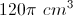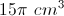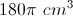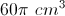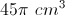Explanation:

The general formula is given by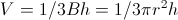, where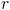= radius and= height.

The diameter is 6 cm, so the radius is 3 cm.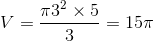### Example Question #1 : Cones

There is a large cone with a radius of 4 meters and height of 18 meters. You can fill the cone with water at a rate of 3 cubic meters every 25 seconds. How long will it take you to fill the cone?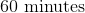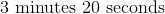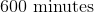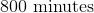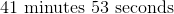Explanation:

First we will calculate the volume of the cone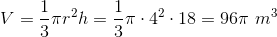Next we will determine the time it will take to fill that volume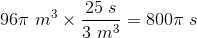We will then convert that into minutes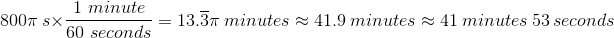### Example Question #1 : Cones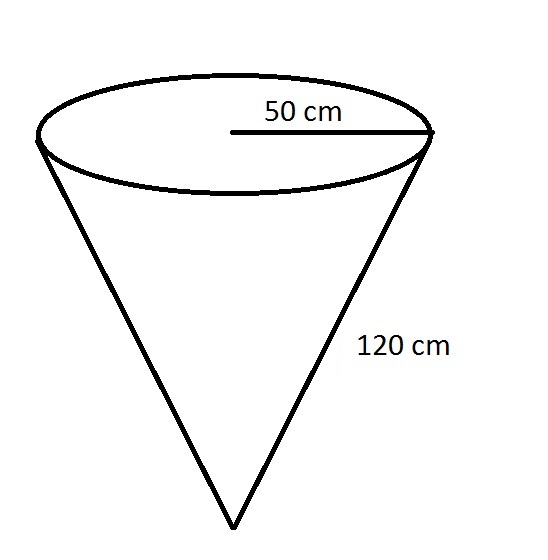Which of these answers comes closest to the volume of the above cone?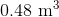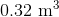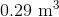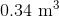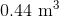Explanation:

The radius and the height of a cone are required in order to find its volume.

The radius is 50 centimeters, which can be converted to meters by dividing by 100: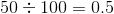meters

The slant height is 120 centimeters, which converts similarly to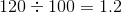meters

To find the height, we need to use the Pythagorean Theorem with the radius 0.5 as one leg and the slant height 1.2 as the hypotenuse of a right triangle, and the heightas the other leg: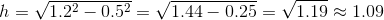meters.

The volume formula can now be used: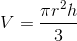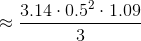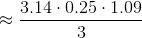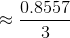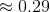cubic meters

### Example Question #1 : How To Find The Volume Of A Cone

What is the volume of a cone with a height of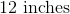and base radius of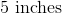?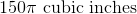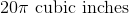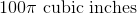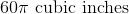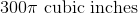Explanation:

The fomula for the volume of a cone is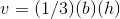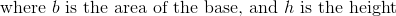Because our radius of the base is, we know that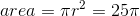.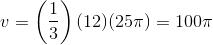.